# Python机器学习方法智能识别亚马逊验证码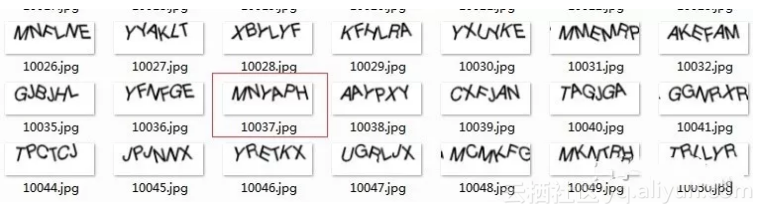1.将原图片作二值化等特殊处理转换得到低像素图片

2.分割出每个字母的图片，并加入到训练库中

3.每个字母图片在训练库中训练

4.将每个字母图片训练后的结果依次组合起来，就是最终验证码

PIL ：图片处理库

scipy ： 科学计算库

●  原图片处理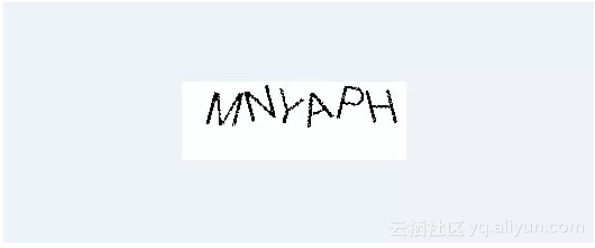im = Image.open(image_file)
 

 
 im = im.convert('P')
 

 
 im_size = im.size
 

 
 new_im = Image.new('P', im_size, 255)
 

 
 im_width = im_size
 
 im_height = im_size
 

 
 for y in range(im_height):
 
 for x in range(im_width):
 
 pixel = im.getpixel((x, y))
 
 if pixel ==0:
 
 new_im.putpixel((x, y), pixel)
  ●  切割图片得到单个字母图片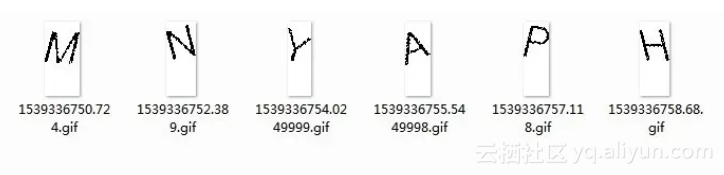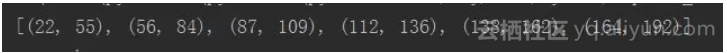def get_x_coord(image) -> '返回切割的x坐标':
 
 image_width = image.size
 
 image_height = image.size
 

 
 crop_list = []
 
 start_pos = 0
 
 is_start_one_char = False
 

 
 for x in range(image_width):
 
 is_black_pos = False
 
 for y in range(image_height):
 
 pixel = image.getpixel((x,y))
 
 if pixel == 0:
 
 if is_start_one_char == False:
 
 start_pos = x
 
 is_black_pos = True
 
 is_start_one_char = True
 
 break
 
 if is_start_one_char== True and is_black_pos == False:
 
 end_pos = x
 
 is_start_one_char = False
 
 crop_list.append((start_pos, end_pos))
 

 
 return crop_list
  ●  单个字母图片加入到训练库中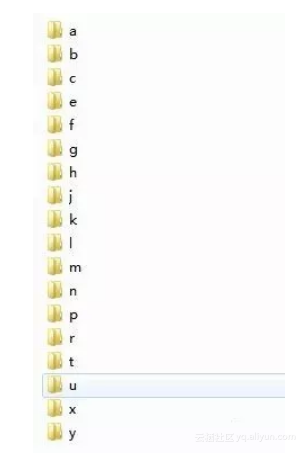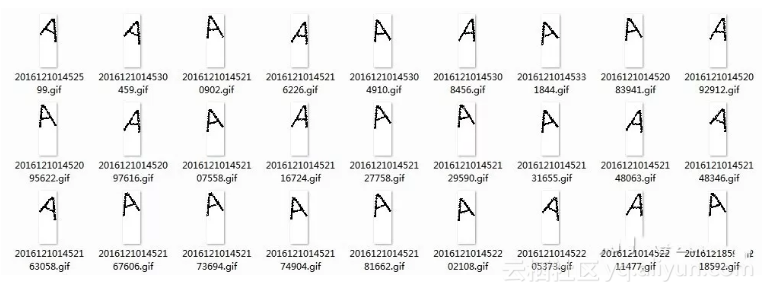●  训练识别单个字母图片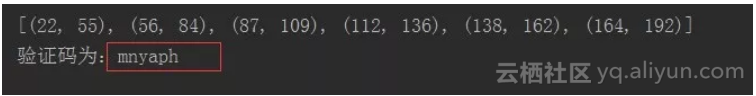match_captcha = []
 
 for crop in crop_list:
 
 crop_im = new_im.crop((crop, 0, crop, im_height)) #（左上x， 左上y， 右下x， 右下y）
 
 filename = 'e:/crop/' + str(time.time()) + '.gif'
 
 # crop_im.save(filename)
 

 
 all_result = [] #单个切片的所有字母的相似性
 

 
 remove_letter = ['d', 'i', 'o', 'q', 's', 'v', 'w', 'z']
 
 for letter in list(set(string.ascii_lowercase)- set(remove_letter)):
 

 
 refer_image_dir = r'E:\training_library\%s' % letter
 

 
 for refer_image in os.listdir(refer_image_dir):
 
 refer_im = Image.open(os.path.join(refer_image_dir, refer_image))
 

 
 crop_list = list(crop_im.getdata())
 
 refer_list = list(refer_im.getdata())
 
 min_count = min(len(crop_list), len(refer_list))
 

 
 result = 1 - spatial.distance.cosine(crop_list[:min_count-1], refer_list[:min_count-1])
 
 all_result.append({'letter' : letter, 'result' : result})
 

 
 match_letter = max(all_result, key=lambda x: x['result']).get('letter')
 
 match_captcha.append(match_letter)
 

 
 print('验证码为：{0}'.format(''.join(match_captcha)))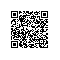Python中文社区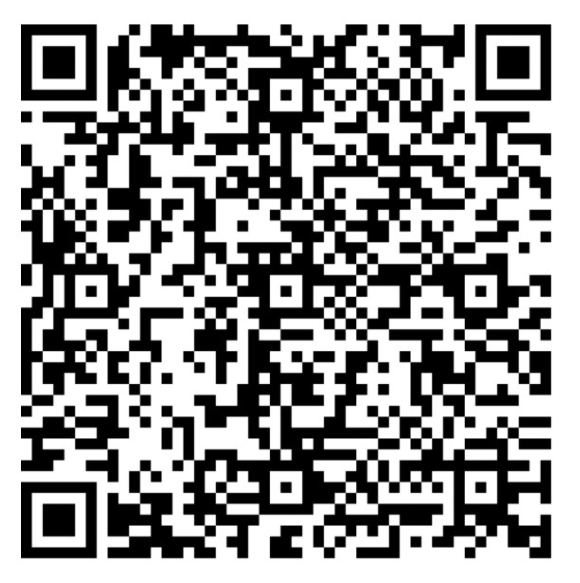使用钉钉扫一扫加入圈子
+ 订阅